# Comparing and ordering numbers up to millions#### All in One Place

Everything you need for JC, LC, and college level maths and science classes.#### Learn with Ease

We’ve mastered the national curriculum so that you can revise with confidence.#### Instant Help

0/2
##### Intros
###### Lessons
1. Introduction to Comparing and Ordering Whole Numbers up to Millions:
2. How to compare two large whole numbers up to millions place values
3. How to order two large whole number up to millions place values
0/9
##### Examples
###### Lessons
1. Comparing values of digits for two multi-digit whole numbers
Fill in each box with the value of each digit for both numbers. Draw a circle around the bigger number.
1. 96,868 or 97,868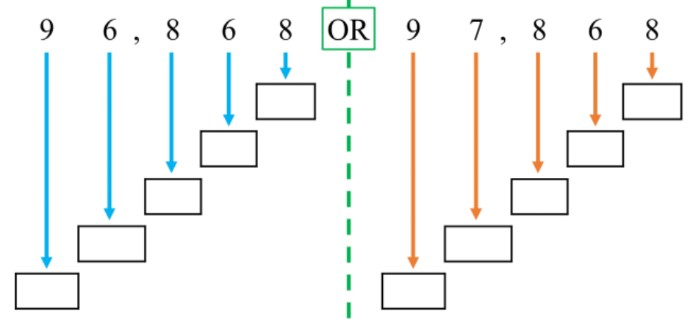2. 3,521,470 or 3,506,841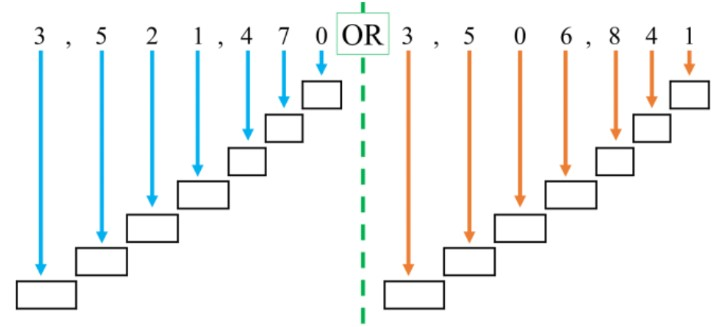2. Comparing two multi-digit numbers up to millions
Compare the numbers. Write the greater than (>) or less than (<) symbol in the blank.
1. 86,109 ___ 86,108
2. 7,934,084 ___ 7,925,152
3. 416,527,938 ___ 426,819,357
3. Ordering multi-digit numbers up to millions: least to greatest
Order the numbers from least to greatest. Use the less than symbol (<).
1. 13 956, 13 967, 13 945
2. 5 283 519, 5 287 472, 5 280 236
4. Ordering multi-digit numbers up to millions: greatest to least
Order the numbers from greatest to least. Use the greater than symbol (>).
1. 637 259, 639 248, 642 117
2. 13 586 214, 22 685 134, 41 865 322
0%
##### Practice
###### Topic Notes

In this lesson, we will learn:

• To compare whole numbers up to millions place values by finding the biggest place value of difference
• To order a list of whole numbers up to millions place values by using a place value table

Notes:

• To compare numbers, look at each place value digit starting on the left (biggest place value)
• Find the biggest place value where the numbers are different to determine which number is bigger or smaller
• Ex. 267,043,359 is bigger than 248,143,358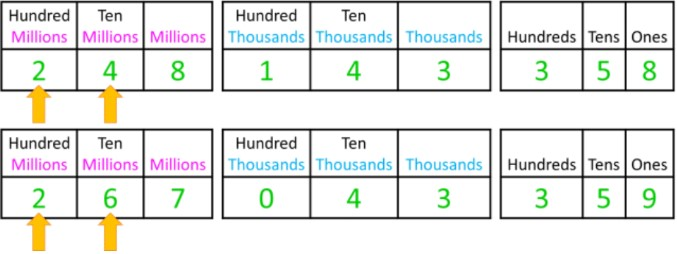• When comparing numbers, we use the symbols: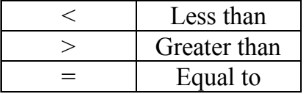• Ex. 267,043,359 is bigger than 248,143,358
Therefore, 267,043,359 > 248,143,358

• There are two ways to order numbers, from:
• Least to greatest (smallest to biggest)
• Or greatest to least (biggest to smallest)

• One tool to help order a list of numbers is by using a place value table
• Ex. Order the numbers from least to greatest: 12 654, 13 465, 9 546, 11 564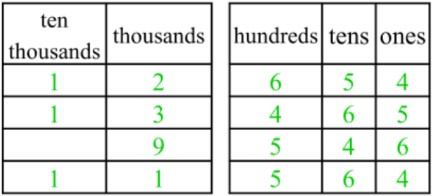• The place value table allows you to compare all place values clearly.
• 9 546 < 11 564 < 12 654 < 13 465